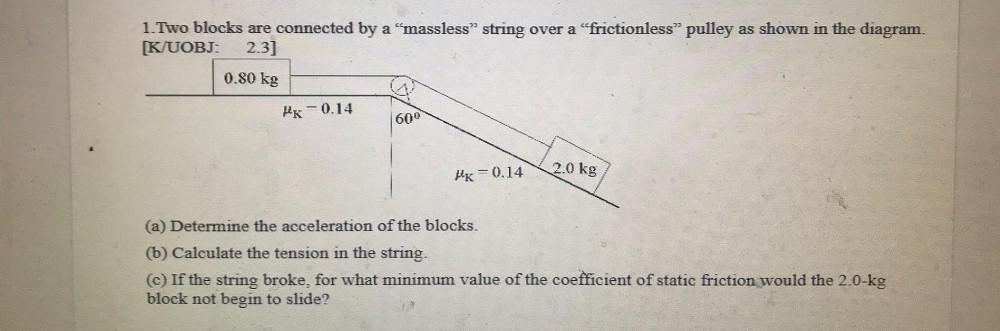Question:

# 1.Two blocks are connected by a “massless" string over a “frictionless” pulley as shown in the diagram. [K/UOBJ: 2.3] 0.80 kg HK1.Two blocks are connected by a “massless" string over a “frictionless” pulley as shown in the diagram. [K/UOBJ: 2.3] 0.80 kg HK -0.14 600 2.0 kg HK = 0.14 (a) Determine the acceleration of the blocks. (b) Calculate the tension in the string. (©) If the string broke, for what minimum value of the coefficient of static friction would the 2.0-kg block not begin to slide?